## Object Detection Using YOLO v2 Deep Learning

This example shows how to train a you only look once (YOLO) v2 object detector.

Deep learning is a powerful machine learning technique that you can use to train robust object detectors. Several techniques for object detection exist, including Faster R-CNN and you only look once (YOLO) v2. This example trains a YOLO v2 vehicle detector using the `trainYOLOv2ObjectDetector` function. For more information, see Object Detection using Deep Learning.

Download a pretrained detector to avoid having to wait for training to complete. If you want to train the detector, set the `doTraining` variable to true.

```doTraining = false; if ~doTraining && ~exist('yolov2ResNet50VehicleExample_19b.mat','file') disp('Downloading pretrained detector (98 MB)...'); pretrainedURL = 'https://www.mathworks.com/supportfiles/vision/data/yolov2ResNet50VehicleExample_19b.mat'; websave('yolov2ResNet50VehicleExample_19b.mat',pretrainedURL); end```

This example uses a small vehicle dataset that contains 295 images. Each image contains one or two labeled instances of a vehicle. A small dataset is useful for exploring the YOLO v2 training procedure, but in practice, more labeled images are needed to train a robust detector. Unzip the vehicle images and load the vehicle ground truth data.

```unzip vehicleDatasetImages.zip data = load('vehicleDatasetGroundTruth.mat'); vehicleDataset = data.vehicleDataset;```

The vehicle data is stored in a two-column table, where the first column contains the image file paths and the second column contains the vehicle bounding boxes.

```% Display first few rows of the data set. vehicleDataset(1:4,:)```
```ans=4×2 table imageFilename vehicle _________________________________ ____________ {'vehicleImages/image_00001.jpg'} {1×4 double} {'vehicleImages/image_00002.jpg'} {1×4 double} {'vehicleImages/image_00003.jpg'} {1×4 double} {'vehicleImages/image_00004.jpg'} {1×4 double} ```
```% Add the fullpath to the local vehicle data folder. vehicleDataset.imageFilename = fullfile(pwd,vehicleDataset.imageFilename);```

Split the data set into a training set for training the detector, and a test set for evaluating the detector. Select 60% of the data for training. Use the rest for evaluation.

```rng(0); shuffledIndices = randperm(height(vehicleDataset)); idx = floor(0.6 * length(shuffledIndices) ); trainingDataTbl = vehicleDataset(shuffledIndices(1:idx),:); testDataTbl = vehicleDataset(shuffledIndices(idx+1:end),:);```

Use `imageDatastore` and `boxLabelDatastore` to create datastores for loading the image and label data during training and evaluation.

```imdsTrain = imageDatastore(trainingDataTbl{:,'imageFilename'}); bldsTrain = boxLabelDatastore(trainingDataTbl(:,'vehicle')); imdsTest = imageDatastore(testDataTbl{:,'imageFilename'}); bldsTest = boxLabelDatastore(testDataTbl(:,'vehicle'));```

Combine image and box label datastores.

```trainingData = combine(imdsTrain,bldsTrain); testData = combine(imdsTest,bldsTest);```

Display one of the training images and box labels.

```data = read(trainingData); I = data{1}; bbox = data{2}; annotatedImage = insertShape(I,'Rectangle',bbox); annotatedImage = imresize(annotatedImage,2); figure imshow(annotatedImage)```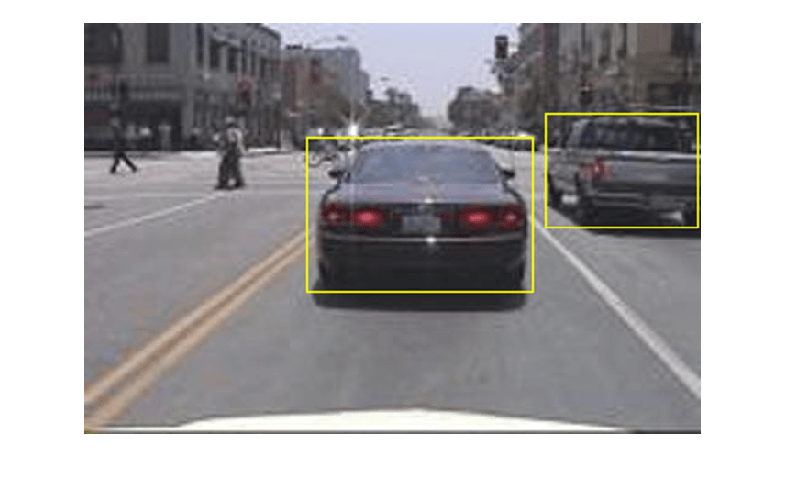### Create a YOLO v2 Object Detection Network

A YOLO v2 object detection network is composed of two subnetworks. A feature extraction network followed by a detection network. The feature extraction network is typically a pretrained CNN (for detials, see Pretrained Deep Neural Networks (Deep Learning Toolbox)). This example uses ResNet-50 for feature extraction. You can also use other pretrained networks such as MobileNet v2 or ResNet-18 can also be used depending on application requirements. The detection sub-network is a small CNN compared to the feature extraction network and is composed of a few convolutional layers and layers specific for YOLO v2.

Use the `yolov2Layers` function to create a YOLO v2 object detection network automatically given a pretrained ResNet-50 feature extraction network. `yolov2Layers` requires you to specify several inputs that parameterize a YOLO v2 network:

• Network input size

• Anchor boxes

• Feature extraction network

First, specify the network input size and the number of classes. When choosing the network input size, consider the minimum size required by the network itself, the size of the training images, and the computational cost incurred by processing data at the selected size. When feasible, choose a network input size that is close to the size of the training image and larger than the input size required for the network. To reduce the computational cost of running the example, specify a network input size of [224 224 3], which is the minimum size required to run the network.

`inputSize = [224 224 3];`

Define the number of object classes to detect.

`numClasses = width(vehicleDataset)-1;`

Note that the training images used in this example are bigger than 224-by-224 and vary in size, so you must resize the images in a preprocessing step prior to training.

Next, use `estimateAnchorBoxes` to estimate anchor boxes based on the size of objects in the training data. To account for the resizing of the images prior to training, resize the training data for estimating anchor boxes. Use `transform` to preprocess the training data, then define the number of anchor boxes and estimate the anchor boxes. Resize the training data to the input image size of the network using the supporting function `preprocessData`.

```trainingDataForEstimation = transform(trainingData,@(data)preprocessData(data,inputSize)); numAnchors = 7; [anchorBoxes, meanIoU] = estimateAnchorBoxes(trainingDataForEstimation, numAnchors)```
```anchorBoxes = 7×2 145 122 81 76 160 132 41 34 63 62 103 97 33 23 ```
```meanIoU = 0.8630 ```

For more information on choosing anchor boxes, see Estimate Anchor Boxes From Training Data (Computer Vision Toolbox™) and Anchor Boxes for Object Detection.

Now, use `resnet50` to load a pretrained ResNet-50 model.

`featureExtractionNetwork = resnet50;`

Select `'activation_40_relu'` as the feature extraction layer to replace the layers after `'activation_40_relu'` with the detection subnetwork. This feature extraction layer outputs feature maps that are downsampled by a factor of 16. This amount of downsampling is a good trade-off between spatial resolution and the strength of the extracted features, as features extracted further down the network encode stronger image features at the cost of spatial resolution. Choosing the optimal feature extraction layer requires empirical analysis.

`featureLayer = 'activation_40_relu';`

Create the YOLO v2 object detection network.

`lgraph = yolov2Layers(inputSize,numClasses,anchorBoxes,featureExtractionNetwork,featureLayer);`

You can visualize the network using `analyzeNetwork` or Deep Network Designer from Deep Learning Toolbox™.

If more control is required over the YOLO v2 network architecture, use Deep Network Designer to design the YOLO v2 detection network manually. For more information, see Design a YOLO v2 Detection Network.

### Data Augmentation

Data augmentation is used to improve network accuracy by randomly transforming the original data during training. By using data augmentation you can add more variety to the training data without actually having to increase the number of labeled training samples.

Use `transform` to augment the training data by randomly flipping the image and associated box labels horizontally. Note that data augmentation is not applied to the test data. Ideally, test data should be representative of the original data and is left unmodified for unbiased evaluation.

`augmentedTrainingData = transform(trainingData,@augmentData);`

Read the same image multiple times and display the augmented training data.

```% Visualize the augmented images. augmentedData = cell(4,1); for k = 1:4 data = read(augmentedTrainingData); augmentedData{k} = insertShape(data{1},'Rectangle',data{2}); reset(augmentedTrainingData); end figure montage(augmentedData,'BorderSize',10)```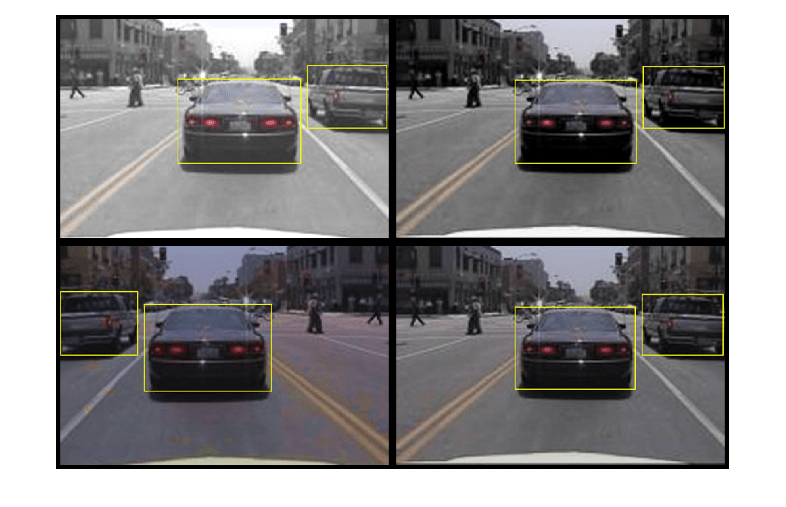### Preprocess Training Data

Preprocess the augmented training data to prepare for training.

`preprocessedTrainingData = transform(augmentedTrainingData,@(data)preprocessData(data,inputSize));`

`data = read(preprocessedTrainingData);`

Display the image and bounding boxes.

```I = data{1}; bbox = data{2}; annotatedImage = insertShape(I,'Rectangle',bbox); annotatedImage = imresize(annotatedImage,2); figure imshow(annotatedImage)```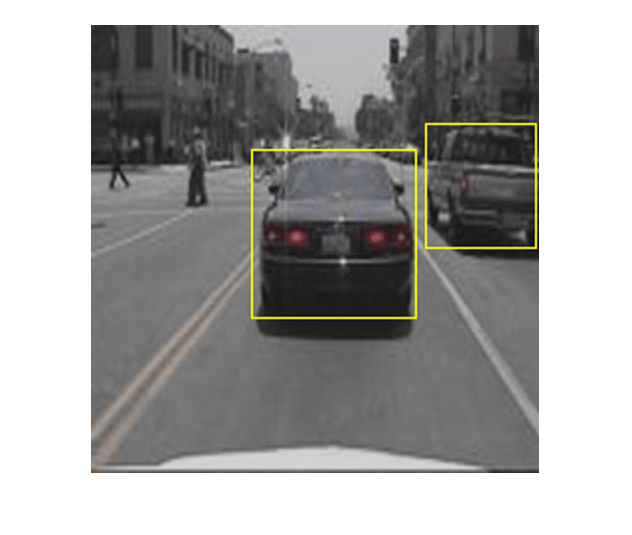### Train YOLO v2 Object Detector

Use `trainingOptions` to specify network training options. Set `'CheckpointPath'` to a temporary location. This enables the saving of partially trained detectors during the training process. If training is interrupted, such as by a power outage or system failure, you can resume training from the saved checkpoint.

```options = trainingOptions('sgdm', ... 'MiniBatchSize', 16, .... 'InitialLearnRate',1e-3, ... 'MaxEpochs',20,... 'CheckpointPath', tempdir, ... 'Shuffle','never');```

Use `trainYOLOv2ObjectDetector` function to train YOLO v2 object detector if `doTraining` is true. Otherwise, load the pretrained network.

```if doTraining % Train the YOLO v2 detector. [detector,info] = trainYOLOv2ObjectDetector(preprocessedTrainingData,lgraph,options); else % Load pretrained detector for the example. pretrained = load('yolov2ResNet50VehicleExample_19b.mat'); detector = pretrained.detector; end```

This example was verified on an NVIDA™ Titan X GPU with 12 GB of memory. If your GPU has less memory, you may run out of memory. If this happens, lower the `'MiniBatchSize'` using the `trainingOptions` function. Training this network took approximately 7 minutes using this setup. Training time varies depending on the hardware you use.

As a quick test, run the detector on one test image. Make sure you resize the image to the same size as the training images.

```I = imread(testDataTbl.imageFilename{1}); I = imresize(I,inputSize(1:2)); [bboxes,scores] = detect(detector,I);```

Display the results.

```I = insertObjectAnnotation(I,'rectangle',bboxes,scores); figure imshow(I)```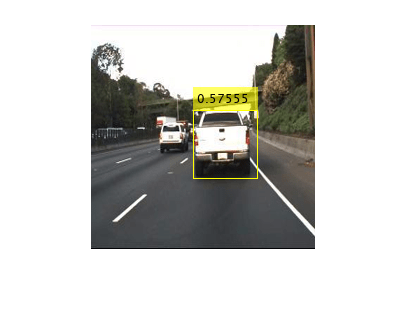### Evaluate Detector Using Test Set

Evaluate the trained object detector on a large set of images to measure the performance. Computer Vision Toolbox™ provides object detector evaluation functions to measure common metrics such as average precision (`evaluateDetectionPrecision`) and log-average miss rates (`evaluateDetectionMissRate`). For this example, use the average precision metric to evaluate performance. The average precision provides a single number that incorporates the ability of the detector to make correct classifications (precision) and the ability of the detector to find all relevant objects (recall).

Apply the same preprocessing transform to the test data as for the training data. Note that data augmentation is not applied to the test data. Test data should be representative of the original data and be left unmodified for unbiased evaluation.

`preprocessedTestData = transform(testData,@(data)preprocessData(data,inputSize));`

Run the detector on all the test images.

`detectionResults = detect(detector, preprocessedTestData);`

Evaluate the object detector using average precision metric.

`[ap,recall,precision] = evaluateDetectionPrecision(detectionResults, preprocessedTestData);`

The precision/recall (PR) curve highlights how precise a detector is at varying levels of recall. The ideal precision is 1 at all recall levels. The use of more data can help improve the average precision but might require more training time. Plot the PR curve.

```figure plot(recall,precision) xlabel('Recall') ylabel('Precision') grid on title(sprintf('Average Precision = %.2f',ap))```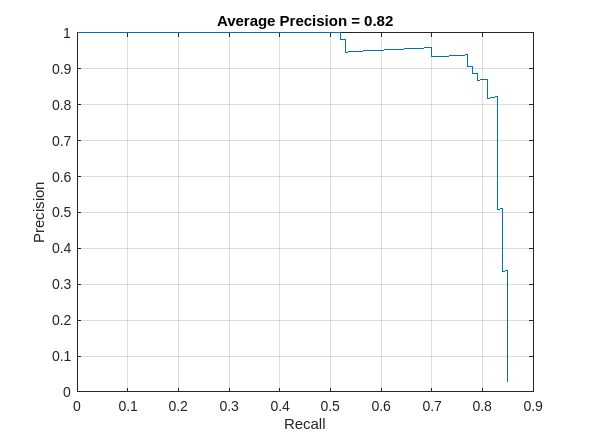### Code Generation

Once the detector is trained and evaluated, you can generate code for the `yolov2ObjectDetector` using GPU Coder™. See Code Generation for Object Detection by Using YOLO v2 (GPU Coder) example for more details.

### Supporting Functions

```function B = augmentData(A) % Apply random horizontal flipping, and random X/Y scaling. Boxes that get % scaled outside the bounds are clipped if the overlap is above 0.25. Also, % jitter image color. B = cell(size(A)); I = A{1}; sz = size(I); if numel(sz)==3 && sz(3) == 3 I = jitterColorHSV(I,... 'Contrast',0.2,... 'Hue',0,... 'Saturation',0.1,... 'Brightness',0.2); end % Randomly flip and scale image. tform = randomAffine2d('XReflection',true,'Scale',[1 1.1]); rout = affineOutputView(sz,tform,'BoundsStyle','CenterOutput'); B{1} = imwarp(I,tform,'OutputView',rout); % Apply same transform to boxes. [B{2},indices] = bboxwarp(A{2},tform,rout,'OverlapThreshold',0.25); B{3} = A{3}(indices); % Return original data only when all boxes are removed by warping. if isempty(indices) B = A; end end function data = preprocessData(data,targetSize) % Resize image and bounding boxes to the targetSize. scale = targetSize(1:2)./size(data{1},[1 2]); data{1} = imresize(data{1},targetSize(1:2)); data{2} = bboxresize(data{2},scale); end```

### References

 Redmon, Joseph, and Ali Farhadi. "YOLO9000: Better, Faster, Stronger." 2017 IEEE Conference on Computer Vision and Pattern Recognition (CVPR). IEEE, 2017.

﻿